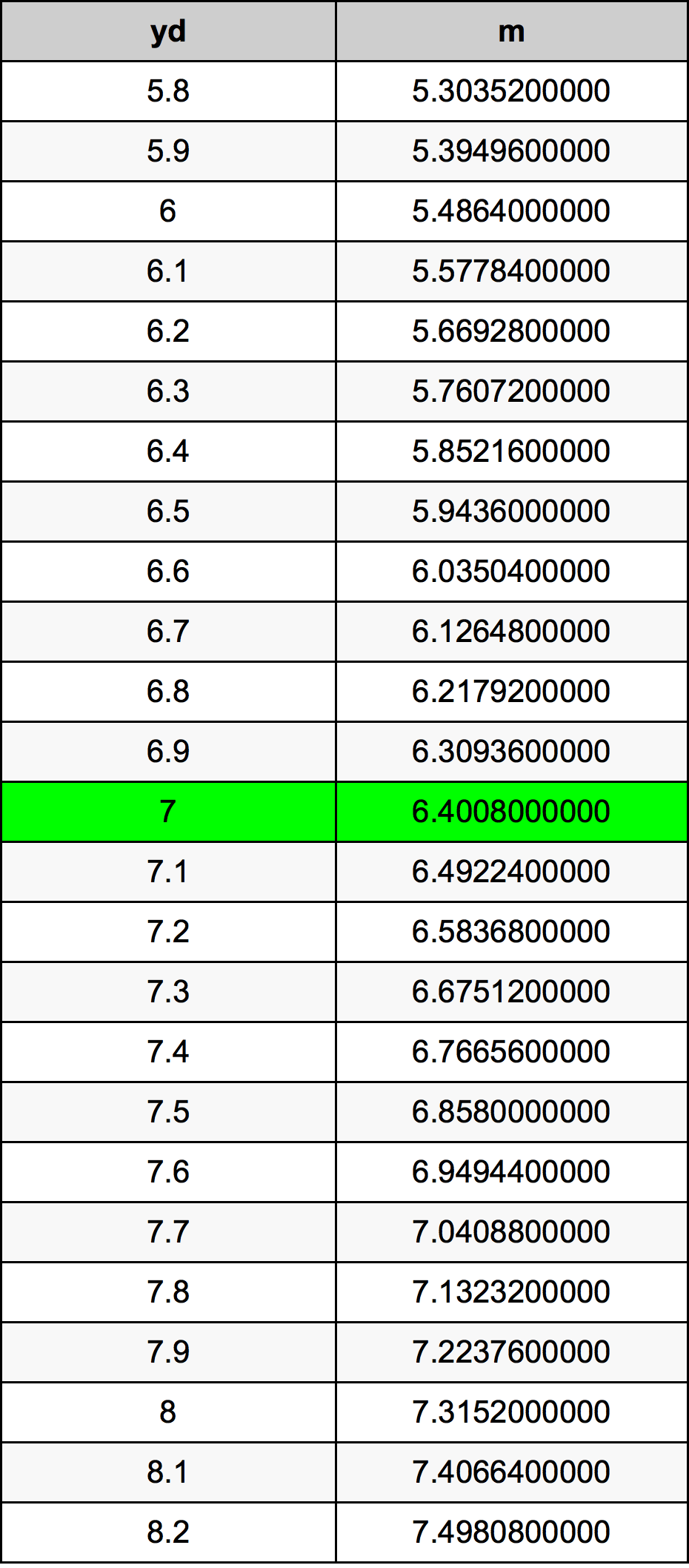Yards To Meters

# 7 yd to m7 Yards to Meters

yd
=
m

## How to convert 7 yards to meters?

 7 yd * 0.9144 m = 6.4008 m 1 yd
A common question is How many yard in 7 meter? And the answer is 7.6552930884 yd in 7 m. Likewise the question how many meter in 7 yard has the answer of 6.4008 m in 7 yd.

## How much are 7 yards in meters?

7 yards equal 6.4008 meters (7yd = 6.4008m). Converting 7 yd to m is easy. Simply use our calculator above, or apply the formula to change the length 7 yd to m.

## Convert 7 yd to common lengths

UnitUnit of length
Nanometer6400800000.0 nm
Micrometer6400800.0 µm
Millimeter6400.8 mm
Centimeter640.08 cm
Inch252.0 in
Foot21.0 ft
Yard7.0 yd
Meter6.4008 m
Kilometer0.0064008 km
Mile0.0039772727 mi
Nautical mile0.0034561555 nmi

## What is 7 yards in m?

To convert 7 yd to m multiply the length in yards by 0.9144. The 7 yd in m formula is [m] = 7 * 0.9144. Thus, for 7 yards in meter we get 6.4008 m.

## 7 Yard Conversion Table## Alternative spelling

7 Yards to Meters, 7 Yards in Meters, 7 Yard to Meter, 7 Yard in Meter, 7 yd to Meter, 7 yd in Meter, 7 Yard to m, 7 Yard in m, 7 yd to m, 7 yd in m, 7 Yard to Meters, 7 Yard in Meters, 7 yd to Meters, 7 yd in Meters